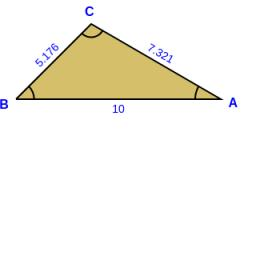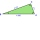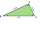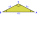# Angles

In the triangle ABC, the ratio of angles is: a:b = 4: 5. The angle c is 36°. How big are the angles a, b?

a =  64
b =  80

### Step-by-step explanation:

a=4/5•b
a+b+36 = 180

5a-4b = 0
a+b = 144

a = 64
b = 80

Our linear equations calculator calculates it.Did you find an error or inaccuracy? Feel free to write us. Thank you!

Showing 1 comment:Math Lover
Can you help me with this (The drawing will be provided. HINT. Use the difference of squares formula a- = (a - b)(a + b). Let AABC be a right-angled triangle with the right angle in A and base BC 50 •6 meters. Let AD be the beight correspond- ing to BC. II AB 30 •6 meters and BD= 18•6 meters, find: AC , DC, AD)Tips to related online calculators
Check out our ratio calculator.

#### You need to know the following knowledge to solve this word math problem:

We encourage you to watch this tutorial video on this math problem:

## Related math problems and questions:

• TrianglesFind out whether given sizes of the angles can be interior angles of a triangle: a) 23°10',84°30',72°20' b) 90°,41°33',48°37' c) 14°51',90°,75°49' d) 58°58',59°59',60°3'
• MO Z7–I–6 2021In the triangle ABC, point D lies on the AC side and point E on the BC side. The sizes of the angles ABD, BAE, CAE and CBD are 30°, 60°, 20° and 30°, respectively. Find the size of the AED angle.
• In a 2In a thirteen sided polygon, the sum of five angles is 1274°, four of the eight angles remaining are equal and the other four are 18° less than each of the equal angles. Find the angles. .
• Depth anglesAt the top of the mountain stands a castle, which has a tower 30 meters high. We see the crossroad in the valley from the top of the tower and heel at depth angles of 32° 50 'and 30° 10'. How high is the top of the mountain above the crossroad
• Chickens and rabbitsIn the yard were chickens and rabbits. Together they had 18 heads and 56 legs. How many chickens and how many rabbits were in the yard?
• Powerplant chimneyFrom the building window at the height of 7.5 m, we can see the top of the factory chimney at an altitude angle of 76° 30 ′. We can see the chimney base from the same place at a depth angle of 5° 50 ′. How tall is the chimney?
• RWYCalculate the opposite direction of the runway 13. Runways are named by a number between 01 and 36, which is generally one tenth of the azimuth of the runway's heading in degrees: a runway numbered 09 points east (90°), runway 18 is south (180°), runway 2
• BisectorsAs shown, in △ ABC, ∠C = 90°, AD bisects ∠BAC, DE⊥AB to E, BE = 2, BC = 6. Find the perimeter of triangle △ BDE.
• Gamma angleFind the magnitude of the gamma angle in triangle ABC if: α = 38° 56 ’and β = 47° 54’.
• The trianglesThe triangles ABC and A'B'C 'are similar with a similarity coefficient of 2. The angles of the triangle ABC are alpha = 35°, beta = 48°. Determine the magnitudes of all angles of triangle A'B'C '.
• Main/central vertexABC is an isosceles triangle with base BC and main vertex A. The angle at vertex A is 18°. What will be the size of the angle at vertex B?
• Elevation anglesFrom the endpoints of the base 240 m long and inclined at an angle of 18° 15 ', the top of the mountain can be seen at elevation angles of 43° and 51°. How high is the mountain?
• Two anglesThe triangles ABC and A'B'C 'are similar. In the ABC triangle, the two angles are 25° and 65°. Explain why in the triangle A'B'C 'is the sum of two angles of 90 degrees.
• Is right triangleOne angle of the triangle is 36° and the remaining two are in the ratio 3:5. Determine whether triangle is a rectangular triangle.
• Internal anglesThe ABCD is an isosceles trapezoid, which holds: |AB| = 2 |BC| = 2 |CD| = 2 |DA|: On its side BC is a K point such that |BK| = 2 |KC|, on its side CD is the point L such that |CL| = 2 |LD|, and on its side DA the point M is such that | DM | = 2 |MA|. Dete
• As shownAs shown, in △ ABC, ∠C = 90°, AD bisects ∠BAC, DE⊥AB to E, BE = 2, BC = 6, then the perimeter of △ BDE
• Outer anglesThe outer angle of the triangle ABC at the A vertex is 71°40 ' outer angle at the vertex B is 136°50'. What size has the inner triangle angle at the vertex C?